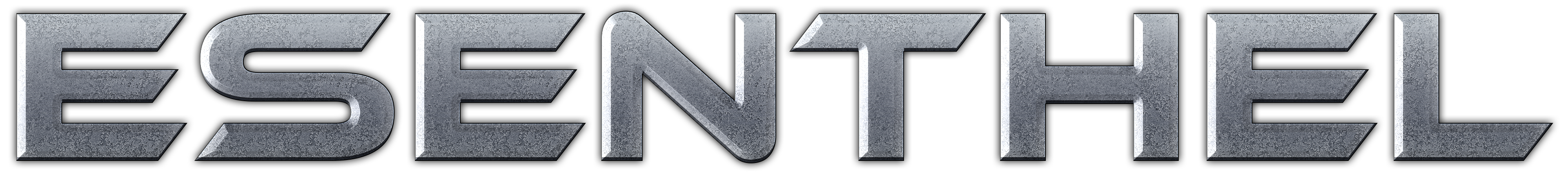About Store Forum Documentation ContactEdit Heightmap Author Message
fatcoderMember

 Post: #1 Edit Heightmap Is it possible, in code, to edit the heightmap of a world loaded from the World Editor? If possible, has anyone worked this out yet? I've had a poke around the headers and see there is an Edit namespace, but I can't work out how this relates to the world you load through the Game namespace.
12-02-2011 12:18 PM
fatcoderMember

 Post: #2 RE: Edit Heightmap OK, well I'm going to consider that it is not possible since I haven't found a way and nobody else has replied. So I have moved on to trying to create a terrain from scratch by generating my own height maps. Anyway, it is all working well except for when it comes to setting the neighbours for each heightmap. Either I don't understand how this works, or it doesn't work properly. The problem is that the heightmap asks you for its neighbours, but the neightbours don't exist yet as they haven't been created yet. But you can't create all the heightmaps first because they all want their neighbours... it is a chicken or the egg problem. Here is my code. Note that WorldArea is my own custom Area struct that I've created to hold the heightmap, mesh, etc for each area. Code: ```FREPD(x,2)    FREPD(z,2)    {       WorldArea *area = areas.get(VecI2(x,z))->data();       Edit::Heightmap *l=NULL,*r=NULL,*b=NULL, *f=NULL,*lb=NULL,*lf=NULL,*rb=NULL,*rf=NULL;       if(areas.find( VecI2( x - 1, z ) ))          l = &areas.get(VecI2( x - 1, z ))->data()->heightmap;       if(areas.find( VecI2( x + 1, z ) ))          r = &areas.get(VecI2( x + 1, z ))->data()->heightmap;       if(areas.find( VecI2( x, z - 1 ) ))          b = &areas.get(VecI2( x, z - 1 ))->data()->heightmap;       if(areas.find( VecI2( x, z + 1 ) ))          f = &areas.get(VecI2( x, z + 1 ))->data()->heightmap;       if(areas.find( VecI2( x - 1, z - 1 ) ))          lb = &areas.get(VecI2( x - 1, z - 1 ))->data()->heightmap;       if(areas.find( VecI2( x - 1, z + 1 ) ))          lf = &areas.get(VecI2( x - 1, z + 1 ))->data()->heightmap;       if(areas.find( VecI2( x + 1, z - 1 ) ))          rb = &areas.get(VecI2( x + 1, z - 1))->data()->heightmap;       if(areas.find( VecI2( x + 1, z + 1 ) ))          rf = &areas.get(VecI2( x + 1, z + 1 ))->data()->heightmap;       area->heightmap.create(areaSize,0,Materials.ptrRequire("mtrl/ground/0.mtrl"),true,l,r,b,f,lb,lf,rb,rf);       Vec2 pos; SinCos(pos.y, pos.x, 3.9f); pos=pos*16+16;       REPD(x2, area->heightmap.resolution())       REPD(y2, area->heightmap.resolution())       {          Flt d=Dist(Vec2(x2,y2), pos);          area->heightmap.height(x2, y2, BlendGaus(d/10)*0.1f);       }       Build(area,l,r,b,f,lb,lf,rb,rf);    }``` Build() goes on to build the heightmap and set up the mesh and phys for it. It is pretty much a copy of the Build method from the Heightmap tutorial. Here is the result. How am I supposed to set the neighbours for each heightmap. I've tried just about everything and nothing seems to work. I'm starting to think that maybe there is a bug...12-03-2011 11:33 AM
fatcoderMember

 Post: #3 RE: Edit Heightmap That requires the Company license which isn't cheap. I can't spend that much money just to find out how to pass neighbours to heightmaps. This should be a simple question and I'm pretty sure I'm doing it correctly, so that is why I'm wondering if there is something actually wrong with the heightmaps. I'm surprised nobody else has tried to do this and ran into the same problem. I don't understand why you have to pass the neighbours when creating a heightmap and then also when building it. If you are creating 4 heightmaps for example that are all neighbours, how can you pass neighbours to the first one when the other three don't exist yet???
12-04-2011 02:40 AM
Esenthelfatcoder